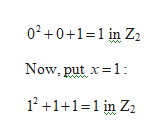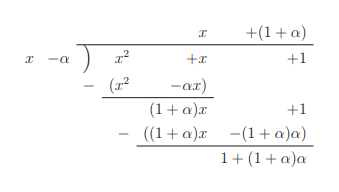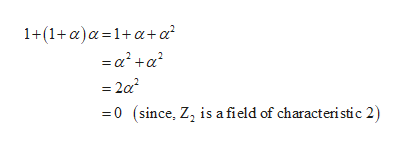Show that x^2 + x + 1 is irreducible over Z_2 and has a zero in some extension field of Z_2 that is a simple extension. This is a problem for abstract algebra that I am struggling with.

Question

Show that x^2 + x + 1 is irreducible over Z_2 and has a zero in some extension field of Z_2 that is a simple extension. This is a problem for abstract algebra that I am struggling with.

Step 1

First, to show the polynomial x2 +x+1 is irreducible over Z2:

Here, recall that a polynomial of degree 2 or 3 is irreducible if and only if it does not have a zero.

Now, since there are only two elements in Z2 that is 0 and 1,

Therefore,

First,

Put x=0 in the polynomial x2 +x+1:help_outlineImage Transcriptionclose02+0+1-1 in Z2 Now, put 1: 12+11 1 in Z2 fullscreen
Step 2

Thus, the polynomial doesn’t have a zero.

Hence, the polynomial x2 +x+1 is irreducible over Z2.

Now, to show that the polynomial x2 +x+1 has a zero in an extension of Z2 :

First let if possible, α be a zero of x2 +x+1 in an extension of Z2

Now, doing long division:help_outlineImage Transcriptionclose+(1a) +1 +x (x2 (1a)a ((1a) (1 a)a) -ax) +1 11a)a fullscreen
Step 3

Now, α will be a zero only if re...help_outlineImage Transcriptionclose1+(1+a)a 1+a+a = = 2a2 0 (since, Z2 is a field of characteristic 2) fullscreen

Want to see the full answer?

See Solution

Want to see this answer and more?

Our solutions are written by experts, many with advanced degrees, and available 24/7

See Solution
Tagged in

Math